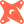Introducing PopSQL + dbt. The first SQL editor with built-in dbt support.Read more →

# How to Compare Arraysin PostgreSQL

The equality operators (`=`, `<>`) do an exact element-by-element comparison.

``````select
array[1,2,3] = array[1,2,4] as compare1, -- arrays are equal
array[1,2,3] <> array[1,2,4] as compare2; -- arrays are not equal``````
`````` compare1 | compare2
----------+----------
f        | t
``````

The ordering operators (`>`, `<`, `>=`, `<=`) also compare each element in an array in order. Results are based on the first different pair of elements, not the sizes of the arrays.

``````select
array[1,2,5] >= array[1,2,4] as compare1,
array[1,2,5] <= array[1,2,4,5] as compare2;``````
`````` compare1 | compare2
----------+----------
t        | f
``````

Then there are the containment operators (`@>`, `<@`). They are casually called "bird operators", well, because `@>` looks like a bird. An array is said to be contained in another array if each of its unique elements is also present in the other array.

``````-- This reads as array['a', 'b', 'c'] contains array['a', 'b', 'b', 'a']
select array['a', 'b', 'c'] @> array['a', 'b', 'b', 'a'] as contains;``````
`````` contains
----------
t
``````
``````-- this reads as array[1, 1, 4] is contained by array[4, 3, 2, 1]
select array[1, 1, 4] <@ array[4, 3, 2, 1] as is_contained_by;``````
`````` is_contained_by
-----------------
t
``````

Lastly, there is the overlap operator (`&&`). Arrays that have elements in common are called overlapping arrays. To check if two arrays overlap, use the `&&` operator:

``````select
array[1, 2] && array[2, 3] as overlap1,
array[1, 2] && array[3, 4] as overlap2;``````
`````` overlap1 | overlap2
----------+----------
t        | f
``````

# Ready for a modern SQL editor?